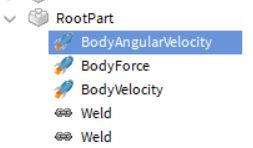# Problems with bodymovers and steering

Currently, I’m using a body gyro to rotate the model and a body velocity to move it.
My problem is in largely two parts.

One, the body velocity doesn’t move towards the orientation of the part (as in, it will continue to move straight forward even if the model is at an angel. https://gyazo.com/ca622088ad38a140929b6c6d22ccfc9b). I had figured the problem was (I think?) due to a body force inside of the root part, however this body force can’t be removed as it keeps the submarine from falling.

Two, how could I use body gyro to rotate the model horizontally? The x axis rotates my model vertically, while the Y axis doesn’t seem to move the model at all (again, probably because of the body force acting upon the y axis), while the z axis makes the model do a barrel roll. Should I be using body angular velocity instead to rotate my model? I had opted to use body gyro as I couldn’t figure out how to move the model in degrees with body angular velocity.

Local script

``````userInputService.InputBegan:connect(function(inputObject, gameProcessedEvent)
if inputObject.KeyCode == Enum.KeyCode.One and not gameProcessedEvent then
print("One")
SubRootPart.BodyVelocity.MaxForce = Vector3.new(10*144000, 0, 10*144000)
SubRootPart.BodyVelocity.Velocity = SubRootPart.CFrame.LookVector * 0
elseif inputObject.KeyCode == Enum.KeyCode.Two and not gameProcessedEvent then
print("Two")
SubRootPart.BodyVelocity.MaxForce = Vector3.new(10*12000, 0, 10*12000)
SubRootPart.BodyVelocity.Velocity = SubRootPart.CFrame.LookVector * 80
elseif inputObject.KeyCode == Enum.KeyCode.Three and not gameProcessedEvent then
print("Three")
SubRootPart.BodyVelocity.MaxForce = Vector3.new(10*24000, 0, 10*24000)
SubRootPart.BodyVelocity.Velocity = SubRootPart.CFrame.LookVector * 80
elseif inputObject.KeyCode == Enum.KeyCode.Four and not gameProcessedEvent then
print("Four")
SubRootPart.BodyVelocity.MaxForce = Vector3.new(10*48000, 10*48000, 10*48000)
SubRootPart.BodyVelocity.Velocity = SubRootPart.CFrame.LookVector * 80
end

if inputObject.KeyCode == Enum.KeyCode.W then
WHeld = true
elseif inputObject.KeyCode == Enum.KeyCode.A then
AHeld = true
elseif inputObject.KeyCode == Enum.KeyCode.S then
SHeld = true
elseif inputObject.KeyCode == Enum.KeyCode.D then
DHeld = true
end
end)

while wait(0.2) do
print(SubRootPart.CFrame.LookVector*80)
if WHeld == true then
SubRootPart.BodyGyro.CFrame = SubRootPart.CFrame * CFrame.Angles(math.rad(1), 0, 0)
elseif AHeld == true then
SubRootPart.BodyGyro.CFrame = SubRootPart.CFrame * CFrame.Angles(0, 0, 0)
elseif SHeld == true then

elseif DHeld == true then
end
end
``````
1 Like

This makes it move in the direction it is pointing, but that only happens when the player presses 1 to 4. You need to constantly update the Velocity to be in line with the object. Since you already have a loop set up, try putting this in the loop:

``````SubRootPart.BodyVelocity.Velocity = SubRootPart.CFrame.LookVector * SubRootPart.BodyVelocity.Velocity.Magnitude
``````

Magnitude isn’t a valid member of body velocity, so I changed it to a number.

For my second problem with body gyro unable to rotate the model horizontally, what would you suggest?
Should I use body angular velocity instead?

Also, occasionally the model would be flung when I run the game, would that just be a problem with the model collisions or something to do with the body movers?

That would make it easier because you can set RelativeTo to World or Attachment0 to get the effect you want.

Could be both. Do you have any other constraints, body movers or such?

I edited my reply from earlier to fix that

If I was to use body angular velocity, how would I rotate the model in degrees?
Also, BodyVelocity.Velocity.Magnitude doesn’t seem to change the velocity as well, what exactly is it supposed to do?

Could be both. Do you have any other constraints, body movers or such?This

``````SubRootPart.BodyVelocity.Velocity = SubRootPart.CFrame.LookVector * SubRootPart.BodyVelocity.Velocity.Magnitude
``````

… only changes the BodyVelocity if the rotation of the sub has changed since it was last updated. It multiplies the direction the sub is facing with the speed that the BodyVelocity was previously set to. That way you get a new velocity that has a new direction but the same speed.

I don’t suggest doing that. I would use an AngularVelocity instead. They’re different things.

You can’t do that directly. Try setting AngularVelocity.AngularVelocity to e.g. (0, 10, 0) when D is held. Make sure you set MaxTorque high enough.

Ah so the body velocity max force isn’t actually the speed of how fast the model is going but how fast the model will accelerate?

The angular velocity vector is changing and I’ve set the max torque to some extremely large number. However nothing is happening with the model and its remaining stationary.

And the problem with the model getting flung at seemingly random times whenever I run the game persists.